# Week 6 Lecture 1

## Curve Fitting

### Polyfit and polyval

The procedure we just outlined for finding a best fit line is so common that MATLAB has a builtin command for it. Instead of finding the derivatives of and setting them to zero, then creating the corresponding linear system and solving it with backslash, you can just use the builtin command polyfit. This command takes three inputs: A vector of x data, a vector of y data and an order. The x and y vectors are just our original data set. We will talk more about the order in the next lecture, but to find a best fit line we should just use 1 for the order. We can therefore try
x = data(1, :);
y = data(2, :);
plot(x, y, 'ko')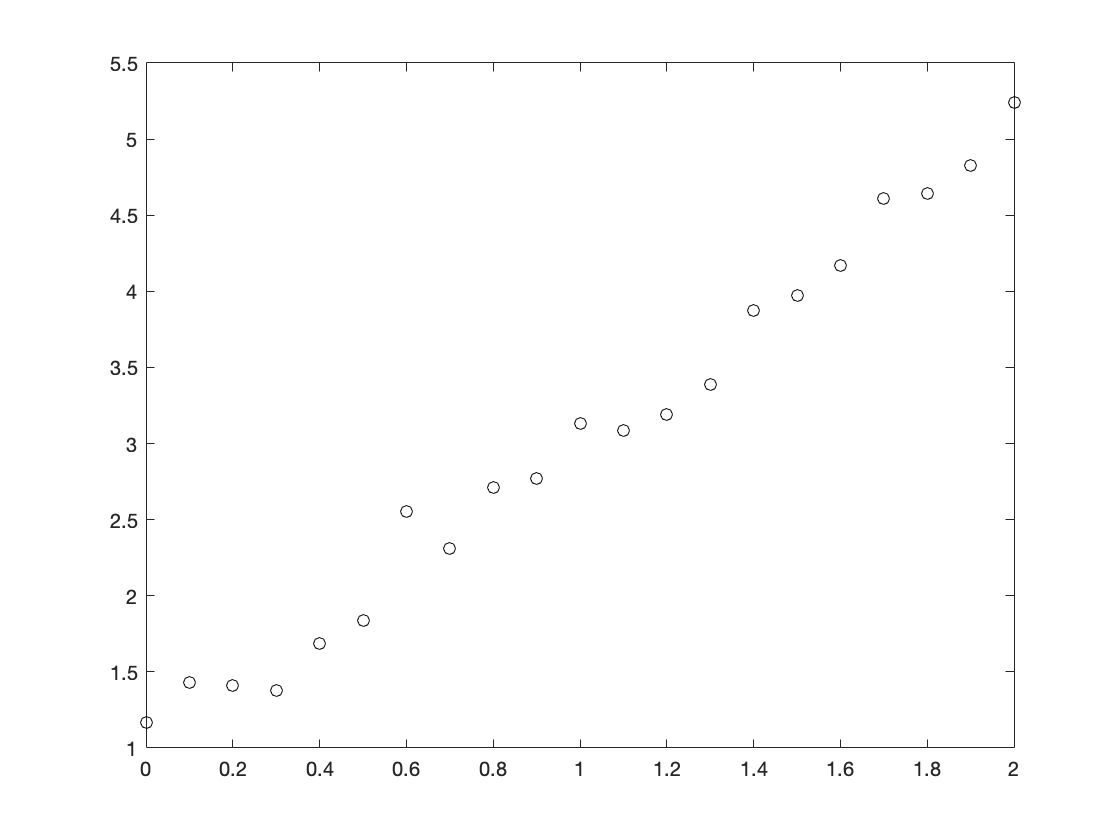coeffs = polyfit(x, y, 1)
coeffs = 1×2
2.0151 1.0038
If we want to graph this best fit line, we can take advantage of another useful builtin function called polyval. This function takes the coefficients of a polynomial (remember, a line is a 1st degree polynomial) and a vector of x-values and then returns a corresponding vector of y-values. We could therefore plot this line with the following code:
xplot = 0:0.001:2;
yplot = polyval(coeffs, xplot);
plot(xplot, yplot, x, y, 'ko')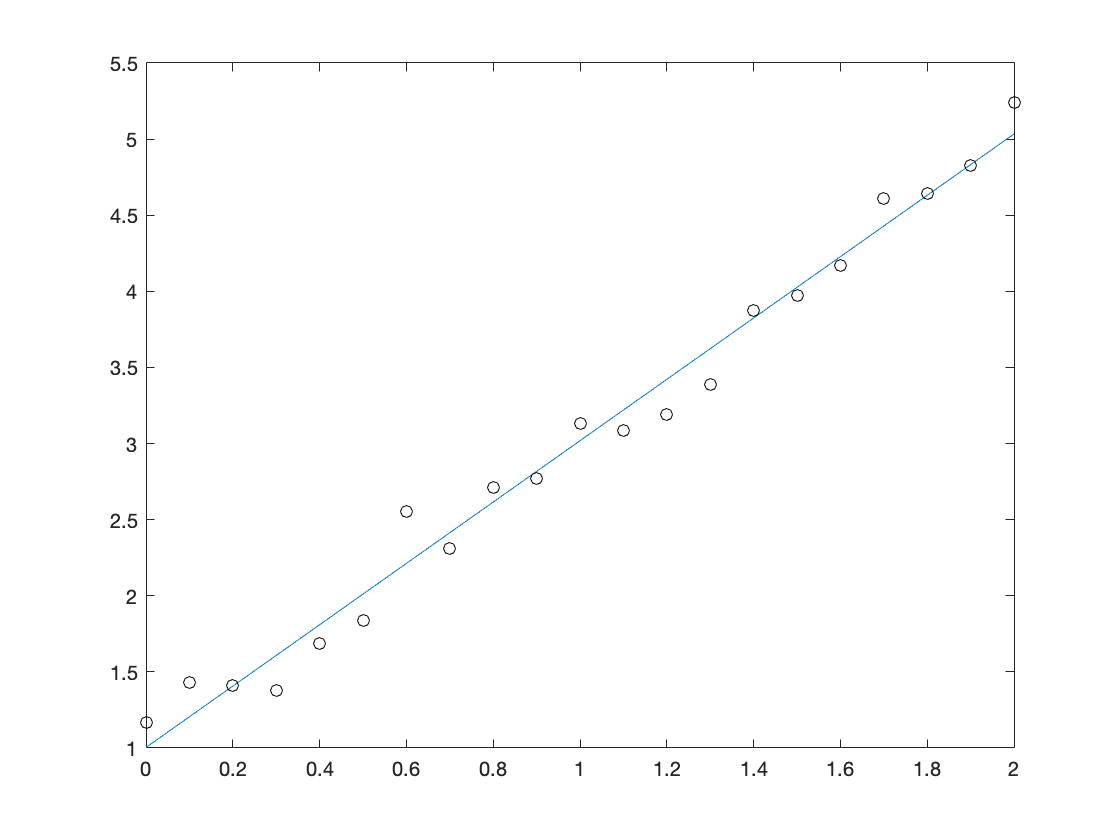The polyval command saves a little bit of typing here and also means that you don't have to remember which order the coefficients from polyfit come in. The polyval command also significantly reduces rounding error when plugging values into higher order polynomials, so it is always better to use polyval than to type out the equation coeffs(1)*xplot + coeffs(2) directly.
In the last section, we talked about how to find the best fit line through a set of data points, either by hand or with the builtin command polyfit. The process that we outlined there can easily be extended to other formulas. In general, if you have a formula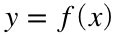that you think fits your data, the RMS error for this fit is defined asIf f has parameters (such as the m and b from our linear equation) then we can minimize E by taking its derivative with respect to each parameter and setting them equal to zero.
Unfortunately, the resulting system of equations is not usually linear and will often be impossible to solve by hand. It turns out that if f is an nth degree polynomial (such as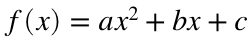or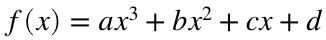) then we get an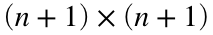linear system of equations, which we could solve with backslash just like before. For most other functions f, the resulting system will be nonlinear and we will have to resort to some other method. This means that we will almost always resort to MATLAB commands to find best fit curves. For some special types of best fit functions, there are builtin MATLAB commands that will find best fit curves for us. For other curves, we can use fminsearch to minimize the RMS error directly. In any case, the curve we find that minimizes the RMS error will be called the best fit curve for our data set. Remember that our definition of "best" depends both on the choice of error function (we will always choose RMS error unless I specify otherwise, but other versions are possible) and on the choice of function. That is, the best fit line and the best fit quadratic are not really being measured on the same scale. We will talk more about the ramifications of this issue in the next section.

### Higher order polynomial fits and overfitting

The polyfit command doesn't just find linear fits. It can actually compute the best fit polynomial of any order. For example, we could find the best fit parabola (i.e., the best fit curve of the form) of the data from last lecture with the code
x = data(1, :);
y = data(2, :);
coeffs2 = polyfit(x, y, 2)
coeffs2 = 1×3
0.1957 1.6237 1.1277
The numbers in coeffs2 are the coefficients a, b and c that give the smallest root-mean-square error with our data set. We can plot this to see if it gives a reasonable fit:
xplot = 0:0.001:2;
yplot = polyval(coeffs2, xplot);
plot(xplot, yplot, 'b', x, y, 'ko')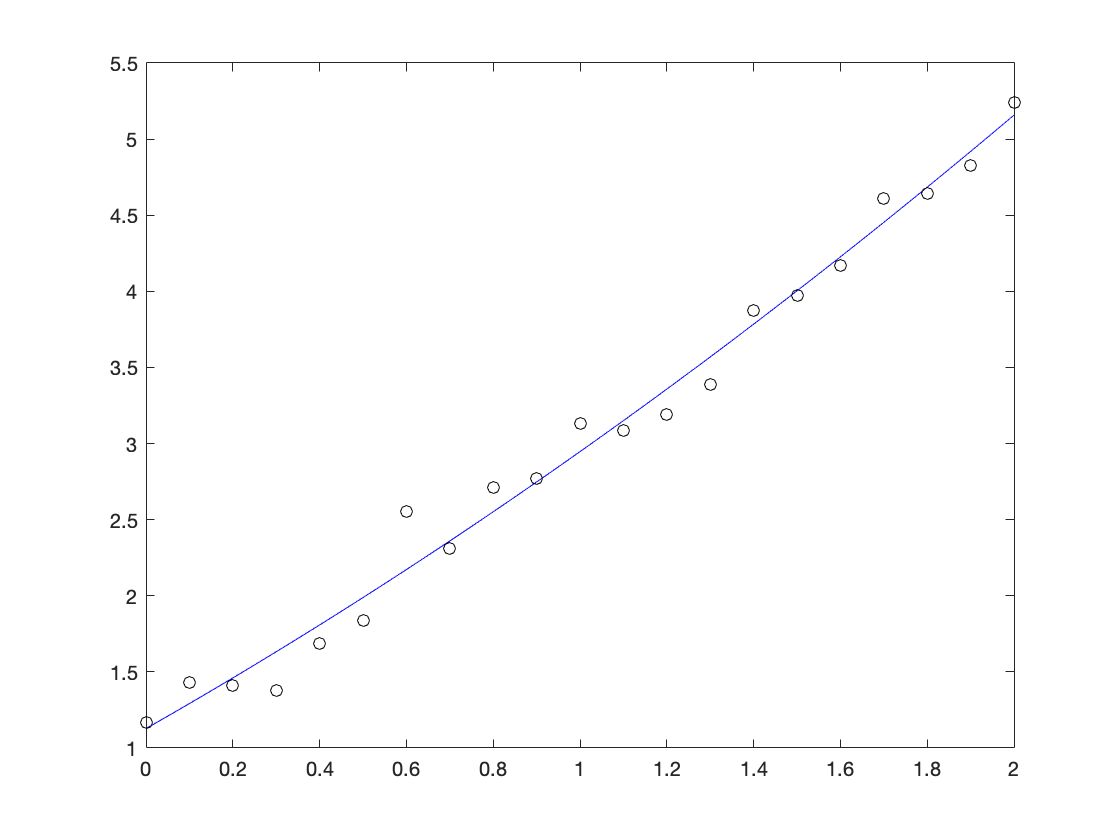It appears to be slightly better than our best fit line from the last one, but it is difficult to say for sure. We could try finding the RMS error to compare:
yhat = polyval(coeffs2, x);
E2 = sqrt(1/length(x)*sum((yhat - y).^2))
E2 = 0.1468
We got a value of approximately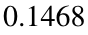, which is smaller than the error we got from our line. In some sense, this means that the quadratic fits our data better than the line. However, one should view this "improvement" with some trepidation. The problem is that increasing the order automatically decreases our error. To see why, imagine what would happen if our line were already the best possible fit. That is, suppose that no other curve could possible come closer to our data points than the best fit line we already found. In that case, we could just choose the quadratic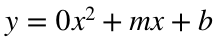and it would be just as good as our line. If the line were anything less than perfect then we could choose some (potentially very small) a in order to improve the fit. This is a problem.
To see why this is bad, you need to know how I came up with the original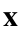and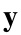. I used the formula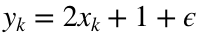, where ϵ was a small random number. (In particular, I used normally distributed random numbers with standard deviation 0.16.) Suppose that I generated one more data point at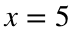.
x_new = 5;
y_new = 2*x_new + 1 + 0.16*randn(1);
Let's add this new point to our plot and see which best fit curve most accurately predicted it. (I reproduced the coefficiencts for our best fit line in coeffs, and coeffs2 holds the coefficients for our best fit quadratic.)
coeffs = polyfit(x, y, 1);
xplot = 0:0.001:5.2;
plot(xplot, polyval(coeffs, xplot), 'b', xplot, polyval(coeffs2, xplot), 'r', x, y, 'ko', x_new, y_new, 'ko')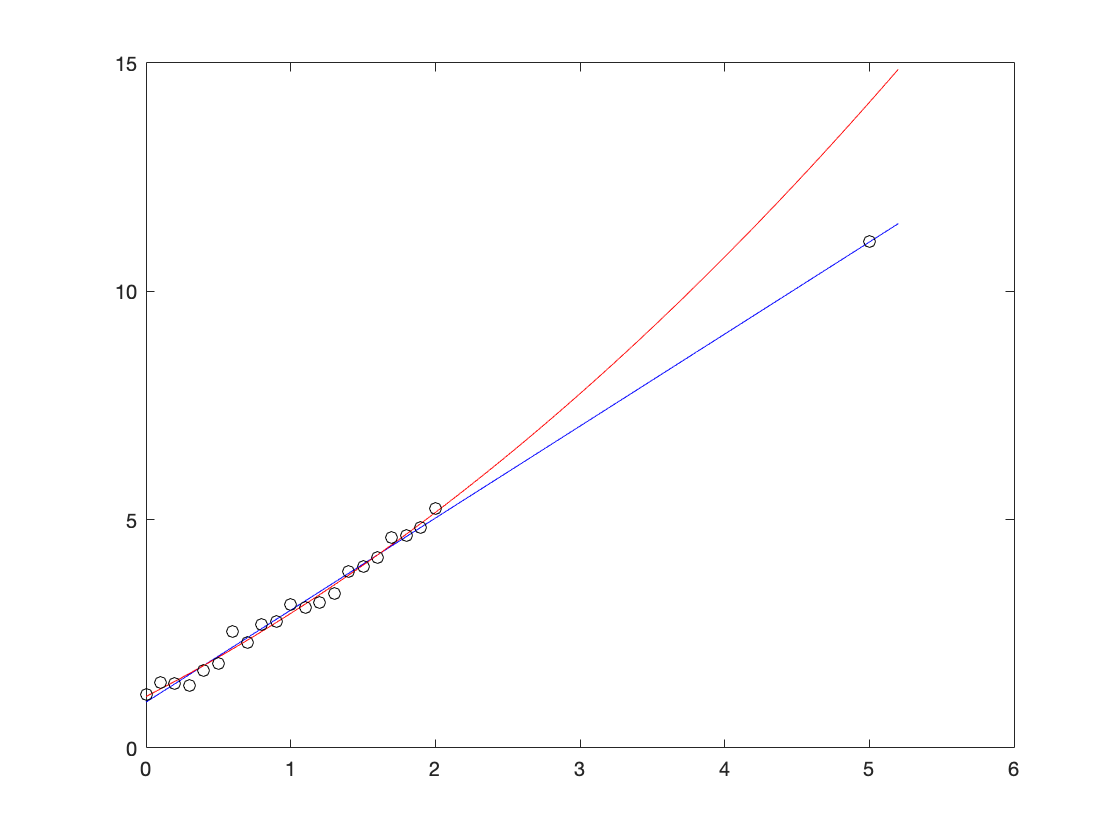Because the new point is random, you will see a different final point every time you run this code, but it will essentially always be much closer to the line (in blue) than to the quadratic (in red). Since our data really did come from a line (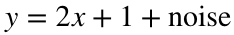), any curve with an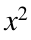term will be worse than the original at predicting new data points. In essence, theterm is capturing patterns in the noise from the original data set that don't really matter, not telling us something important about our data. Using a higher order polynomial like this (or using any curve with too many parameters in it) is called overfitting. The main problem with overfitting is that your curve will be worse at predicting new data, even though it matches the existing data better. That is, if I make a new data point using the same formula as I did originally (or measure a new data point using the same measurement process) then it is likely to be very close to the original best fit line, but there is no reason to hope that it is close to the overfitted curve.

### Extreme overfitting

We can carry this idea of overfitting to an extreme. Notice that our original data set has 21 data points. Likewise, a 20th order polynomial has 21 coefficients. This means that we can find a 20th order polynomial that perfectly fits our data set. (We will just use the polyfit command, but the optional videos show a slicker method called "Lagrange polynomials".)
coeffs = polyfit(x, y, 20);
Warning: Polynomial is badly conditioned. Add points with distinct X values, reduce the degree of the polynomial, or try centering and scaling as described in HELP POLYFIT.
xplot = 0:0.01:2;
yplot = polyval(coeffs, xplot);
plot(xplot, yplot, 'b', x, y, 'ko')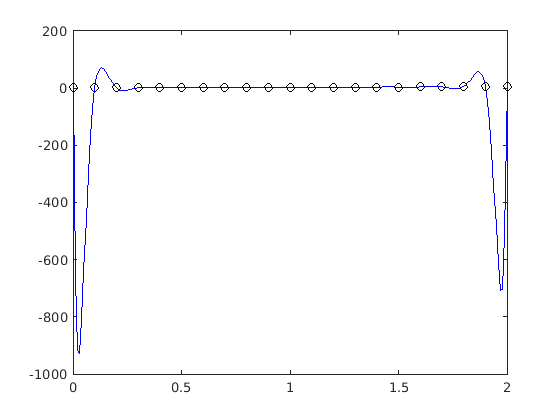What happened? We hit every single data point, but this graph certainly doesn't describe any trends in our data. The phenomenon where this best fit polynomial oscillates wildly is called "Runge's phenomenon", or the more whimsical "polynomial wiggle", and it means that we should essentially never use high order polynomial fits like this.
There is actually another problem with high order fits, although it is hard to see with this data set. High order polynomials naturally lead to serious rounding errors, which make any calculations involving these polynomials (including just graphing) highly unstable. That is why MATLAB gives the warning "Polynomial is badly conditioned." If you try this on a larger data set (such as the one in the next homework) you will see a dramatic example of rounding error in action. The polyval command helps with this issue because it evaluates polynomials in a smarter order, but there is no way to completely eliminate the problem. You should always use polyval to evaluate high order polynomials.

### Fitting nonlinear data

While it is true that overfitting is an important risk to keep in mind, and that the risk grows as we add more parameters to the curve we are fitting, it is also sometimes a good idea to try to fit a more complicated curve to our data. As an example, consider the data in the file Week6_data2.csv.
x = data(1, :);
y = data(2, :);
n = length(x);
plot(x, y, 'ko')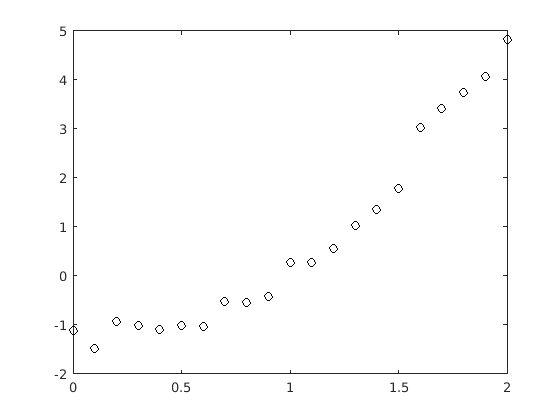This data looks roughly linear, but there does seem to be a recognizable "curve" in the graph. We could, of course, try to find a best fit line for this data set. Using the same code as in the last lecture, we get the following plot:
coeffs = polyfit(x, y, 1);
xplot = 0:0.01:2;
yplot = polyval(coeffs, xplot);
plot(x, y, 'ko', xplot, yplot, 'b')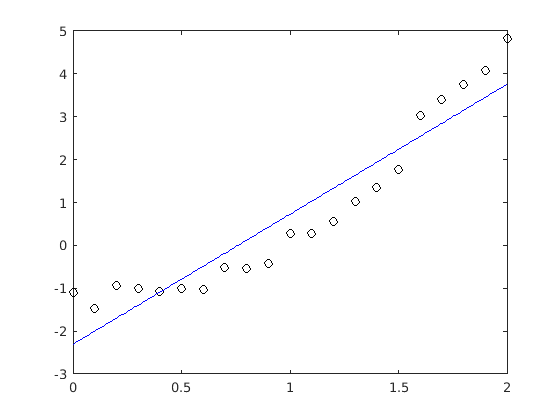This doesn't look like a terrible fit, but we do seem to be systematically off. Our line is too low at the edges and too high in the middle. We can quantify how "far off" our curve is with the root mean square error of this fit.
E = sqrt((1/n)*sum((polyval(coeffs, x) - y).^2))
E = 0.6473
We don't really have anything useful to compare this error to yet, but it's worth noting that this is substantially larger than the linear fit from last lecture. (Normally that is not relevant, but when I made the two data sets I used random numbers with the same standard deviation, which means that if our data really were linear we would expect roughly the same RMS error as before.)
Let's try a higher order polynomial fit.
coeffs2 = polyfit(x, y, 2);
yplot = polyval(coeffs2, xplot);
plot(x, y, 'ko', xplot, yplot, 'r')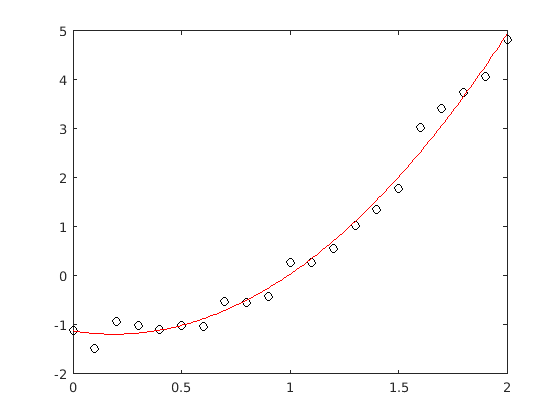At least visually, this seems like a big improvement. We can also check the RMS error to try to quantify how much better it is.
E = sqrt((1/n)*sum((polyval(coeffs2, x) - y).^2))
E = 0.2068
Keep in mind that this error is automatically lower than the error for our best fit line, just because we added an extra parameter to our curve. That said, this is a pretty substantial improvement. The error for our best fit quadratic is less than a third of the error for our best fit line.
What if we keep going? If we got this much improvement out of a quadratic, what will happen if we try a cubic fit?
coeffs3 = polyfit(x, y, 3);
yplot = polyval(coeffs3, xplot);
plot(x, y, 'ko', xplot, yplot, 'g')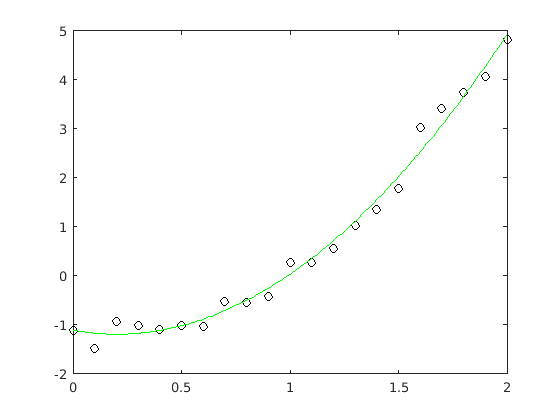This does not look like nearly as much of an improvement as the quadratic. Again, we can quantify the change by finding the RMS error for our new fit.
E = sqrt((1/n)*sum((polyval(coeffs3, x) - y).^2))
E = 0.2067
Once again, the error is automatically lower because we used an extra parameter in our curve. In this case, though, the error is only 0.1% lower instead of nearly 2/3 lower. This tells us that going from a quadratic to a cubic was not a good idea.
These results are no coincidence. When I generated this data, I did so with the formula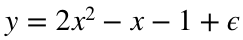, where ϵ was a small random number. This means that a best fit line can't possibly fully describe the process I used to make the data, but a best fit quadratic can. Notice that the coefficients of our best fit quadratic are actually relatively close to correct. Instead of 2, -1 and -1 we got 1.9, -0.7 and -1.1. These are not as accurate as with the previous data set, when we predicted the coefficients of our best fit line to the first decmal place, but they are still reasonably close. As a general rule, if your formula has more parameters, you need more data to accurately predict these parameters. If we had 100 points in our dataset instead of 21, then we would have obtained a much more accurate estimate of our formula.

### Non-polynomial curves

So far, both of our datasets have really come from polynomials. (The first set came from a linear function and the second came from a quadratic function.) In general, we can't actually hope that all of our data will be in such a nice form. As an example, consider the data in Week6_data3.csv.
x = data(1, :);
y = data(2, :);
n = length(x);
plot(x, y, 'ko')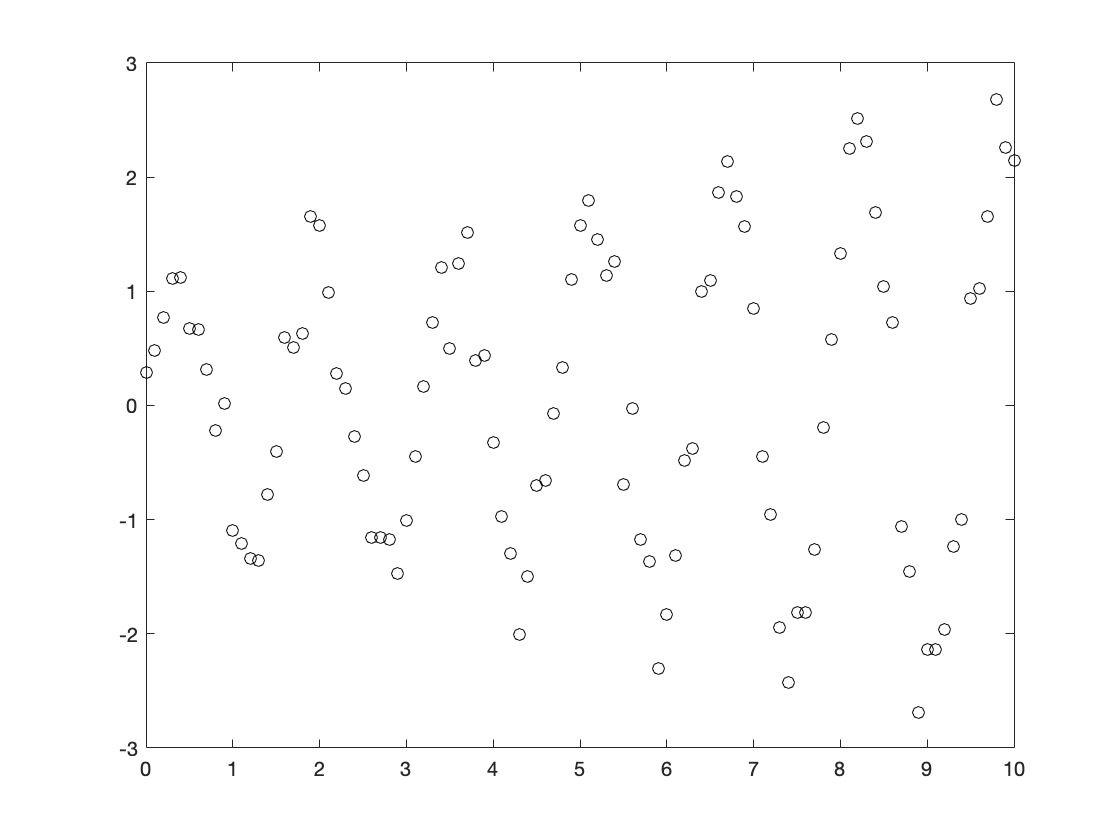At first glance, this data might appear roughly linear. It seems to be centered around the line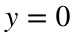with quite a bit of noise. If you squint at the data, though, you might see some more systematic patterns to it. For one thing, the points appear to be getting more spread out as x increases. For another, it looks like there might be a regular oscillation instead of just noise. That is, the points appear to rise and fall at regular intervals. You should be wary of this sort of visual analysis, though. You can always find more patterns if you squint at your data hard enough, but that doesn't mean there is a real underlying pattern.
With all that said, it never hurts to start by finding a linear fit to our dataset. We get the following line and RMS error:
coeffs = polyfit(x, y, 1);
xplot = 0:0.01:10;
yplot = polyval(coeffs, xplot);
plot(x, y, 'ko', xplot, yplot, 'b')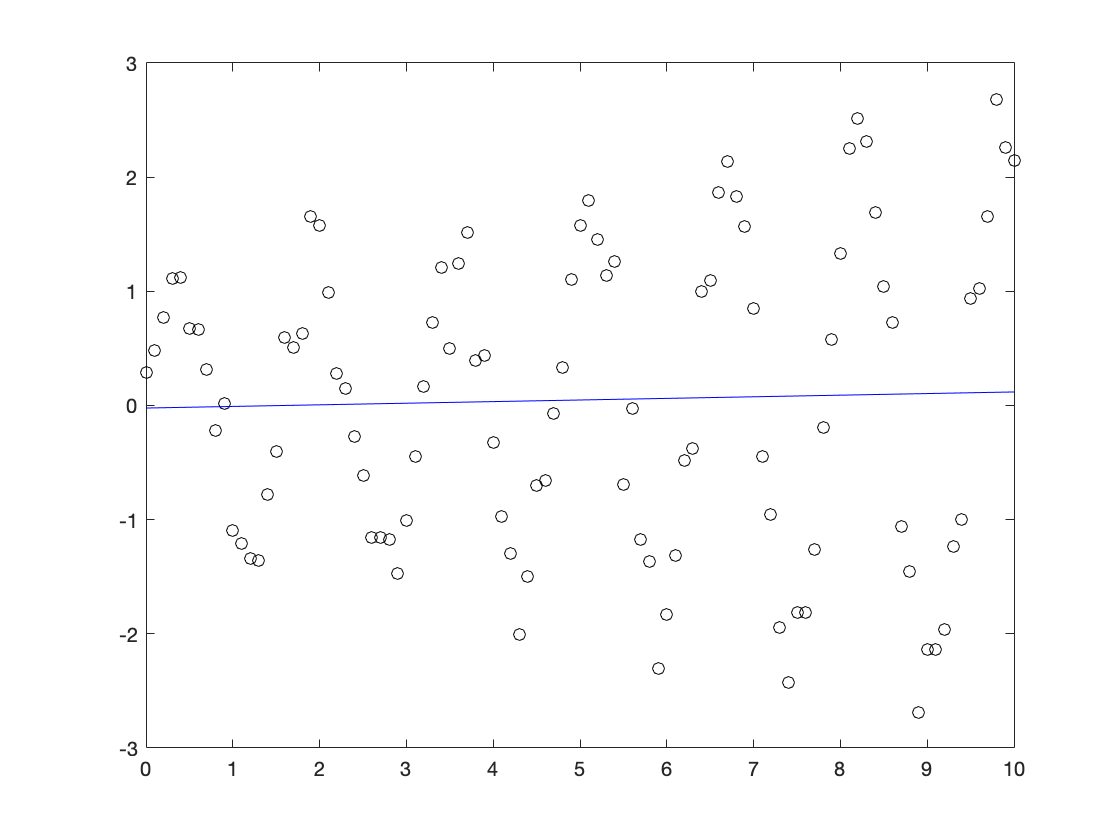#### Rahman notes:

The notes that were passed down show some pitfalls that we should be aware of, and some naive fixes, but I think it might be interesting to be a bit clever about this. So this might be a bit complex, but I think it will pay dividends if anyone goes into any sort of data-related field.
We see that the data seems to look like a sinusoidal with an exponential profile. Let's first tackle the sinusoidal. How do we figure out the period? One way is to look at the troughs. The distance between troughs will give us the period. We did something similar last week.
count = 0;
for i = 1:length(x)-1
if sign(y(i)) ~= sign(y(i+1)) && abs(x(j)-x(i)) > 0.5
count = count + 1;
j = i;
end
end
x_sin = [x(1):0.001:x(end)];
y_sin = sin(x_sin*pi*count/(x(j)-x(1)));
plot(x, y, 'ko', x_sin, y_sin, 'linewidth',4 , 'markersize', 10)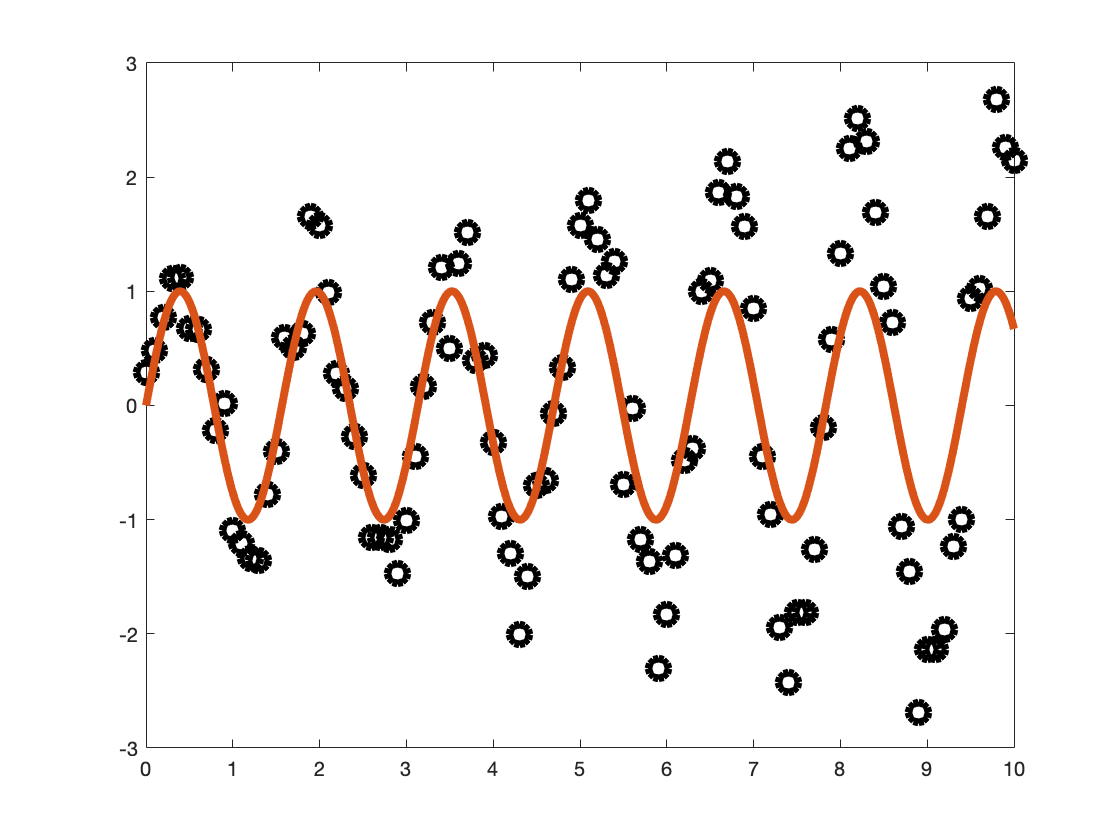x_peaks = zeros(count/2 + 1, 1);
y_peaks = x_peaks;
count = 0;
for i = 1:length(x)-2
if y(i) < y(i+1) && y(i+2) < y(i+1) && abs(x(j)-x(i)) > 0.5 && y(i+1) > 0
count = count + 1;
y_peaks(count) = y(i+1);
x_peaks(count) = x(i+1);
j = i;
end
end
plot(x_peaks, y_peaks, 'o')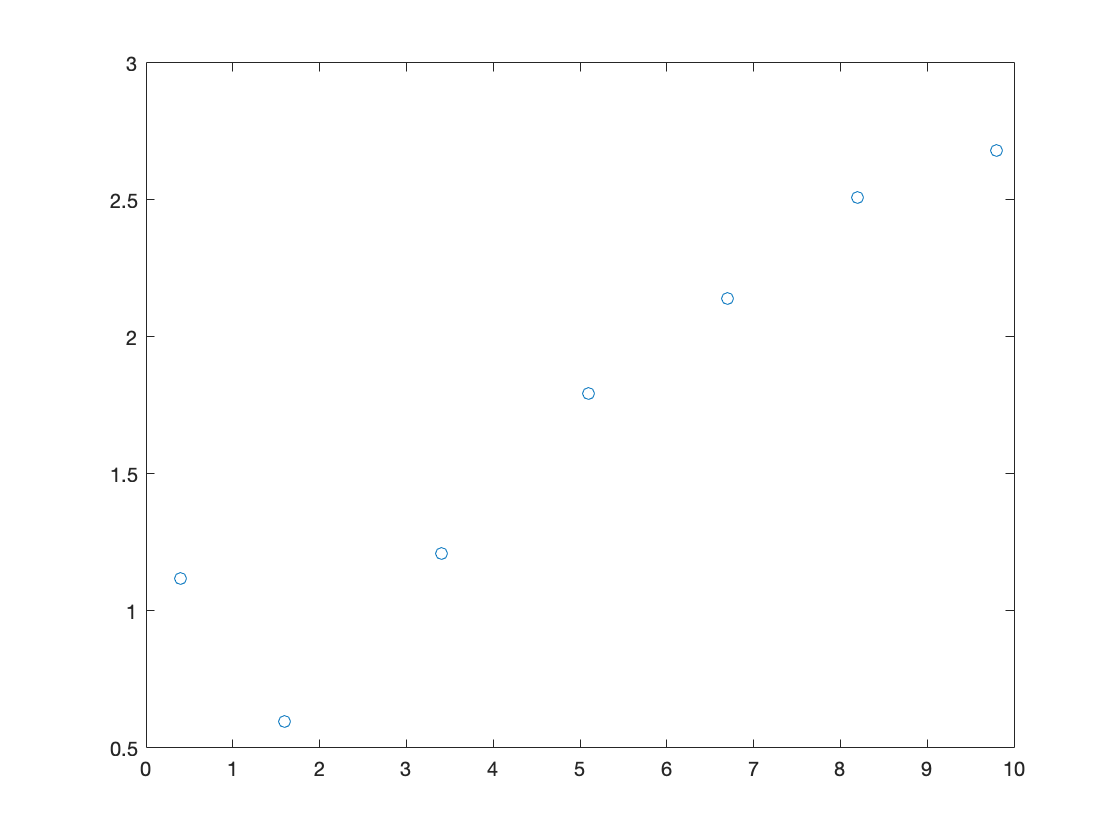coeffs = polyfit(x_peaks, log(y_peaks),1)
coeffs = 1×2
0.1383 -0.2617
x_envelope = [x(1):0.001:x(end)];
y_envelope = exp(coeffs(1)*x_envelope + coeffs(2));
plot(x, y, 'ko', x_envelope, y_envelope, 'linewidth',4 , 'markersize', 10)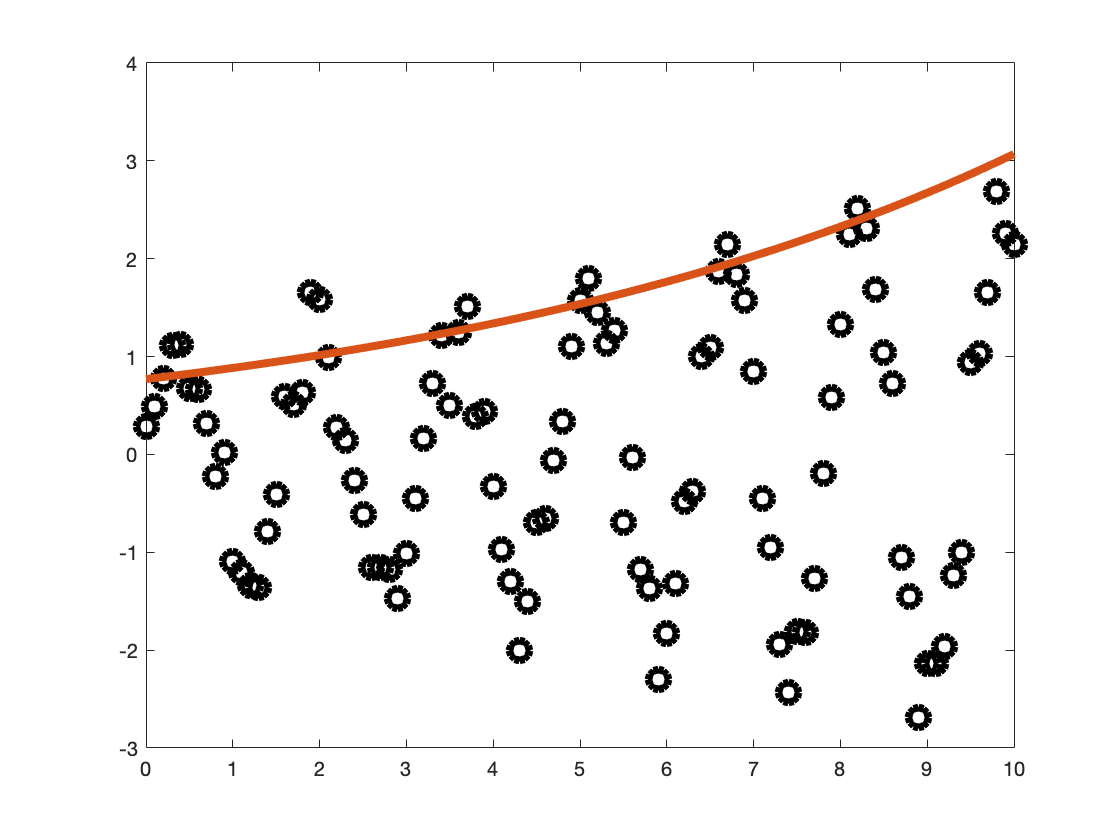plot(x, y, 'ko', x_envelope, y_envelope.*y_sin, 'linewidth',4 , 'markersize', 10)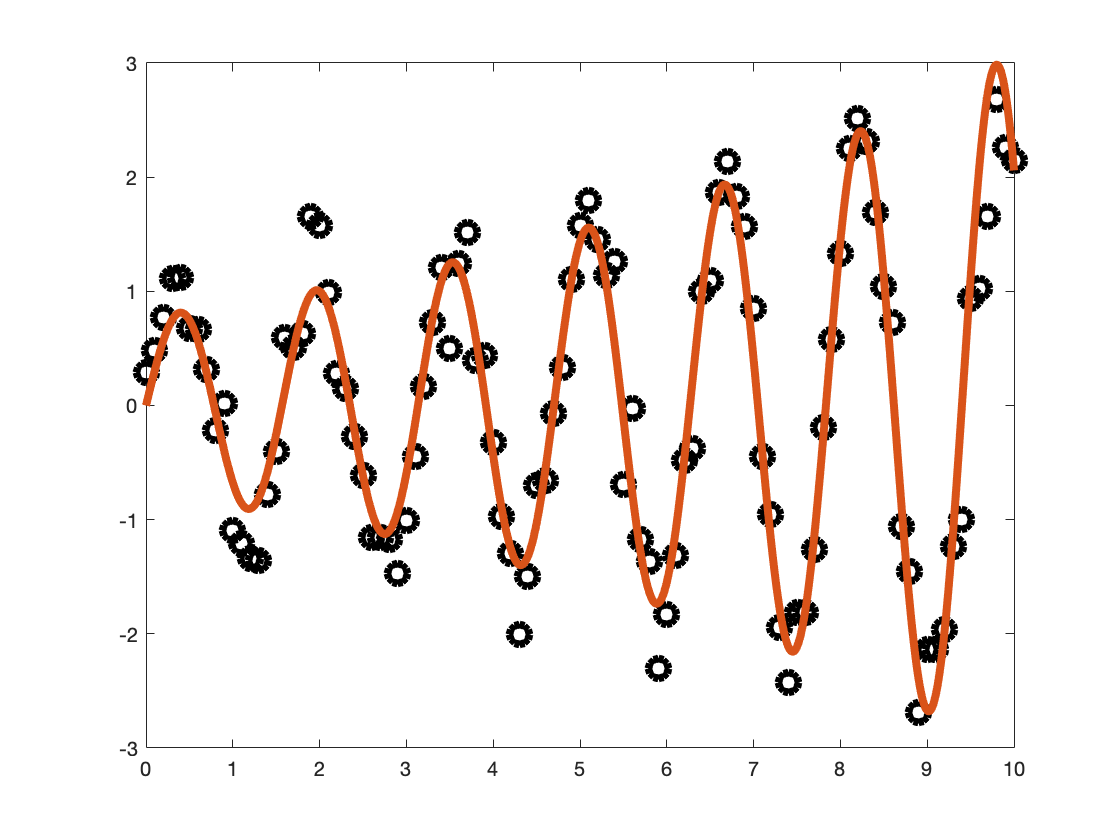### Older notes:

E = sqrt((1/n)*sum((polyval(coeffs, x) - y).^2))
E = 1.3194
This line doesn't look like a particularly good fit, but it is possible that these data really are just random numbers, in which case there is nothing more we can do. The error value doesn't tell us very much because we don't have any other errors to compare to.
We could do the same thing as before and try higher order polynomial fits to see if they do any better. There is a plausible (but entirely wrong) argument for why this might be a good idea: If our data was generated by the formula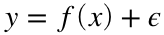for some reasonably nice function f, then the function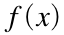should have a Taylor expansion. Maybe if we found high order polynomial fits to our data, they would give us a good approximation to the Taylor expansion of, and therefore a good approximation toitself. We know that it is a bad idea to use too high an order for our fit, but maybe we could safely use a medium order polynomial. It turns out that this is just not how data fitting really works. There are two different issues: First, Taylor approximations rely on a lot of detailed information (higher order derivatives) aboutvery close to one point, while we have lots of data about f at widely spread out points. Second, small errors in our data (and, in practice, all data has small errors) lead to huge changes in these higher order polynomial fits, even if the data is still generated from the same function. As an illustrative example, though, let's try this out:
coeffs2 = polyfit(x, y, 2);
coeffs3 = polyfit(x, y, 3);
coeffs4 = polyfit(x, y, 4);
yplot2 = polyval(coeffs2, xplot);
yplot3 = polyval(coeffs3, xplot);
yplot4 = polyval(coeffs4, xplot);
plot(x, y, 'ko', xplot, yplot2, 'r', xplot, yplot3, 'm', xplot, yplot4, 'g')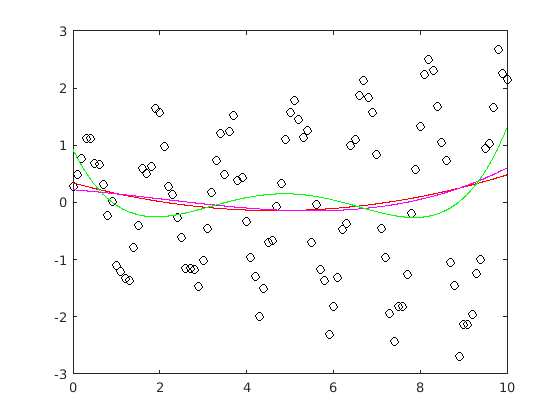As you can see, these do not appear to match the data very well. Maybe we didn't go high enough?
coeffs90 = polyfit(x, y, 90);
Warning: Polynomial is badly conditioned. Add points with distinct X values, reduce the degree of the polynomial, or try centering and scaling as described in HELP POLYFIT.
yplot90 = polyval(coeffs90, xplot);
plot(x, y, 'ko', xplot, yplot90, 'r')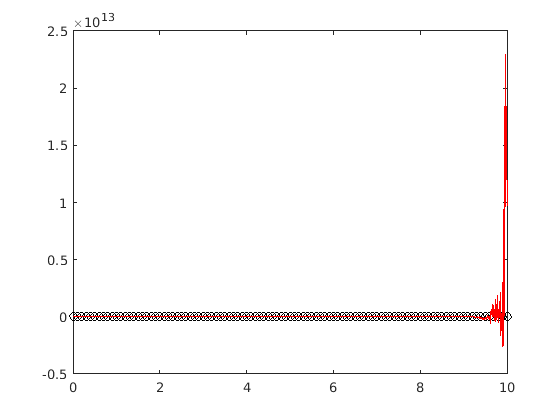This is a good illustration of the problems with high order polynomial fits. You may be able to find a medium order polynomial that appears to be a good fit to the data (in this case, a 20th order polynomial does not look bad at all) but it is entirely by coincidence. The data did not come from a polynomial formula, and we are not really approximating the real formula well with our polynomial fits. In general, this idea of trying higher and higher order polynomials simply doesn't work.
If polynomial fits won't work, then what are we supposed to do? It turns out that there is a way to proceed, but we need more information. In particular, we need to know more about the formula we got our data from. In this case, I made the data myself, so I know that it came from the formula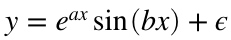, where ϵ was a small random number. This is not a polynomial, but it is a formula with relatively few parameters. That means that we can try to find a best fit curve of the appropriate form. Unfortunately, MATLAB does not have a builtin function "exponential_sine_fit", so we will need to do a little more work than in the case of polynomials.
To start, let's just try a couple examples for a and b.
a = 1;
b = 1;
yplot = exp(a*xplot) .* sin(b*xplot);
plot(x, y, 'ko', xplot, yplot, 'b')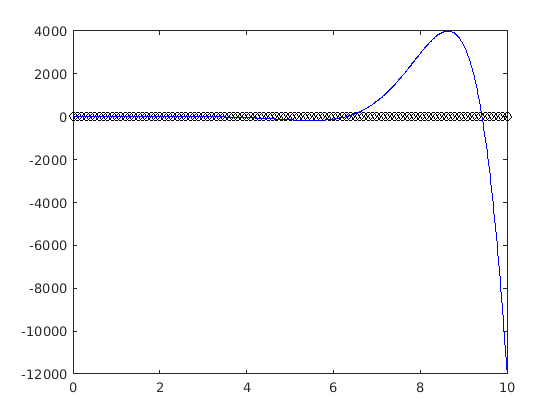This is obviously not a very good fit. We can quantify just how bad it is with the RMS error:
E = sqrt((1/n)*sum((exp(a*x).*sin(b*x) - y).^2))
E = 2.2022e+03
The problem is that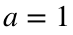and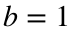was not a very good guess. Let's try again.
a = 0.1;
b = 5;
yplot = exp(a*xplot).*sin(b*xplot);
plot(x, y, 'ko', xplot, yplot, 'b')